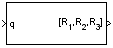Documentation

This is machine translation

Mouseover text to see original. Click the button below to return to the English version of the page.

Quaternions to Rotation Angles

Determine rotation vector from quaternion

Library

Utilities/Axes TransformationsDescription

The Quaternions to Rotation Angles block converts the four-element quaternion vector (q0, q1, q2, q3) into the rotation described by the three rotation angles (R1, R2, R3). The block generates the conversion by comparing elements in the direction cosine matrix (DCM) as a function of the rotation angles. The elements in the DCM are functions of a unit quaternion vector. For example, for the rotation order `z-y-x`, the DCM is defined as:

`$DCM=\left[\begin{array}{lll}\mathrm{cos}\theta \mathrm{cos}\psi \hfill & \mathrm{cos}\theta \mathrm{sin}\psi \hfill & -\mathrm{sin}\theta \hfill \\ \left(\mathrm{sin}\varphi \mathrm{sin}\theta \mathrm{cos}\psi -\mathrm{cos}\varphi \mathrm{sin}\psi \right)\hfill & \left(\mathrm{sin}\varphi \mathrm{sin}\theta \mathrm{sin}\psi +\mathrm{cos}\varphi \mathrm{cos}\psi \right)\hfill & \mathrm{sin}\varphi \mathrm{cos}\theta \hfill \\ \left(\mathrm{cos}\varphi \mathrm{sin}\theta \mathrm{cos}\psi +\mathrm{sin}\varphi \mathrm{sin}\psi \right)\hfill & \left(\mathrm{cos}\varphi \mathrm{sin}\theta \mathrm{sin}\psi -\mathrm{sin}\varphi \mathrm{cos}\psi \right)\hfill & \mathrm{cos}\varphi \mathrm{cos}\theta \hfill \end{array}\right]$`

The DCM defined by a unit quaternion vector is:

`$DCM=\left[\begin{array}{lll}\left({q}_{0}^{2}+{q}_{1}^{2}-{q}_{2}^{2}-{q}_{3}^{2}\right)\hfill & 2\left({q}_{1}{q}_{2}+{q}_{0}{q}_{3}\right)\hfill & 2\left({q}_{1}{q}_{3}-{q}_{0}{q}_{2}\right)\hfill \\ 2\left({q}_{1}{q}_{2}-{q}_{0}{q}_{3}\right)\hfill & \left({q}_{0}^{2}-{q}_{1}^{2}+{q}_{2}^{2}-{q}_{3}^{2}\right)\hfill & 2\left({q}_{2}{q}_{3}+{q}_{0}{q}_{1}\right)\hfill \\ 2\left({q}_{1}{q}_{3}+{q}_{0}{q}_{2}\right)\hfill & 2\left({q}_{2}{q}_{3}-{q}_{0}{q}_{1}\right)\hfill & \left({q}_{0}^{2}-{q}_{1}^{2}-{q}_{2}^{2}+{q}_{3}^{2}\right)\hfill \end{array}\right]$`

From the preceding equation, you can derive the following relationships between DCM elements and individual rotation angles for a ZYX rotation order:

`$\begin{array}{c}\varphi =\text{atan}\left(DCM\left(2,3\right),DCM\left(3,3\right)\right)\\ =\text{atan}\left(2\left({q}_{2}{q}_{3}+{q}_{0}{q}_{1}\right),\left({q}_{0}^{2}-{q}_{1}^{2}-{q}_{2}^{2}+{q}_{3}^{2}\right)\right)\\ \theta =\text{asin}\left(-DCM\left(1,3\right)\right)\\ =\text{asin}\left(-2\left({q}_{1}{q}_{3}-{q}_{0}{q}_{2}\right)\right)\\ \psi =\text{atan}\left(DCM\left(1,2\right),DCM\left(1,1\right)\right)\\ =\text{atan}\left(2\left({q}_{1}{q}_{2}+{q}_{0}{q}_{3}\right),\left({q}_{0}^{2}+{q}_{1}^{2}-{q}_{2}^{2}-{q}_{3}^{2}\right)\right)\end{array}$`

where Ψ is R1, Θ is R2, and Φ is R3.

Parameters

Rotation Order

Specifies the output rotation order for three rotation angles. From the list, select `ZYX`, `ZYZ`, `ZXY`, `ZXZ`, `YXZ`, `YXY`, `YZX`, `YZY`, `XYZ`, `XYX`, `XZY`, or `XZX`. The default is `ZYX`.

Inputs and Outputs

InputDimension TypeDescription

First

4-by-1 quaternion vectorContains the quaternion vector.
OutputDimension TypeDescription

First

3-by-1 vectorContains the rotation angles, in radians.

Assumptions and Limitations

For the 'ZYX', 'ZXY', 'YXZ', 'YZX', 'XYZ', and 'XZY' rotations, the block generates an R2 angle that lies between ±pi/2 radians, and R1 and R3 angles that lie between ±pi radians.

For the 'ZYZ', 'ZXZ', 'YXY', 'YZY', 'XYX', and 'XZX' rotations, the block generates an R2 angle that lies between 0 and pi radians, and R1 and R3 angles that lie between ±pi radians. However, in the latter case, when R2 is 0, R3 is set to 0 radians.

Introduced in R2007b

Aerospace Blockset DocumentationGet trial now